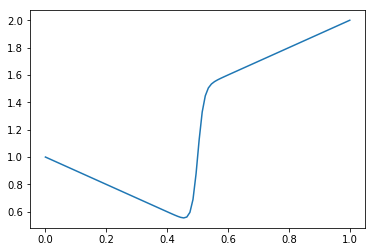Special Sauce¶

Suppose we have a function like the following below.

In :
import numpy as np
import matplotlib.pyplot as plt
%matplotlib inline
In :
def f(x) :
return x * np.tanh(50.0*(x-0.5)) + 1.0

x = np.linspace(0,1,100)
plt.plot(x,f(x));Some parts change slowly (or change uniformly), and some parts change quickly.

Question, how would you set the interval size $\Delta x$ to get an accurate integral using the trapazoid method?

• Answer, use variable width trapazoidsQuestion, how do you know where to put the variable widths?

Question, what if you want adaptive trapazoid widths for any function, even ones you don't know yet?

• That is, for any function that a user might supply.
• How can you make your code figure out where to put more resolution?

• Put points uniformly along the curve of the function.

All of these are pretty complicated, there is a simpler way

• What is the simplest possible application of trapazoid method?
• ...What is the next simplest?
• Now, compare $I_1$ and $I_2$.
• If they give pretty much the same values then we are done.
• If they are different, then do the same 1 and 2 trapazoid comparison in each half of the domain.
• Repeat in each subregion.• This can be done with a recursive algorithm
In :